# How to use the IFS function in Google SheetsThe IF function in Google Sheets helps you categorize data using a simple if-then-else construct. It checks whether a condition in a cell is true or false. But what if you need to incorporate multiple sets of criteria? You might think that nesting IFs is the solution and you’re not wrong!

Nested IF statements are handy but they can generate a very long formula, which can be difficult to work with and lead to errors in your spreadsheet. Fortunately, however, there is a less cumbersome function that you can use — the IFS function.

This takes in a set of expression and value pairs, evaluates them in sequence, and returns the first value whose corresponding expression is TRUE. If no expression evaluates as TRUE, it returns an #N/A! error.

The following flow chart explains the process: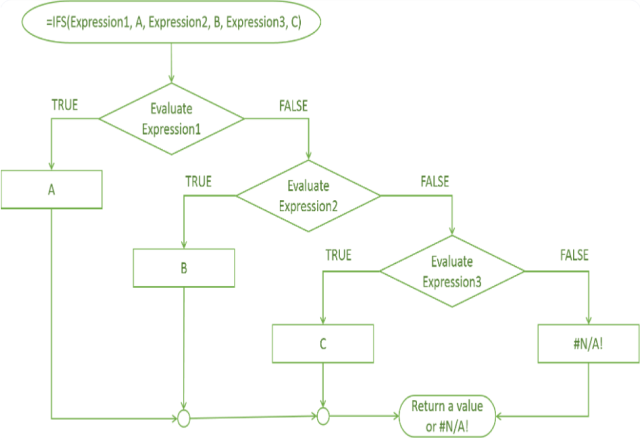### Syntax

=IFS(expression1, value1, [expression2, value2], …)

• expression1 – the first logical expression that Google Sheets evaluates as either TRUE or FALSE
• value1 – the value that the IFS function returns if the corresponding expression1 evaluates as TRUE
• expression2 – the next logical expression that evaluates as either TRUE or FALSE if expression1 evaluates as FALSE
• value2 – the value that returns if expression2 evaluates as TRUE.

Please note that only the first expression and value pair is mandatory. The subsequent pairs are optional.

And remember, the result of the function will be from the first expression that is evaluated as TRUE — subsequent expressions will only be evaluated if the preceding expressions are FALSE.

### How to use the IFS function

In the example below, I have entered the formula =IFS(A2>100,A2&” is greater than 100″,A2<100,A2&” is less than 100″).

I am asking the function to evaluate the numbers 50 and 150 and to tell me whether they are less than or greater than 100.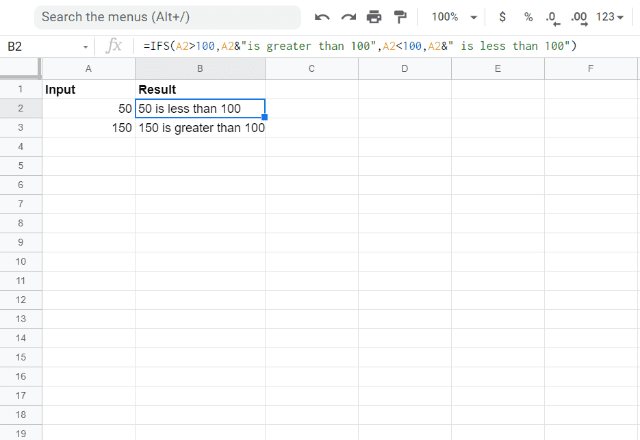The function starts by evaluating the first expression, in this case, 50>100, which is obviously FALSE. So, it moves on to the next expression, 50<100, which is TRUE. Hence it returns the value “50 is less than 100”.

In the second example, in row 3, it is doing the same thing, but the value is now 150. So when the function evaluates the first expression, it finds 150>100 to be TRUE. It then outputs the value “150 is greater than 100”, and does not evaluate the second expression.

What happens if all the expressions in the IFS function evaluate to FALSE? Let’s take a look, with the help of a different example.

In the image below, I have used the IFS function to group people based on their age. The formula used here is =IFS(B2<10,”Kid”,B2<20,”Adolescent”,B2<30,”Young adult”,B2<60,”Middle aged”).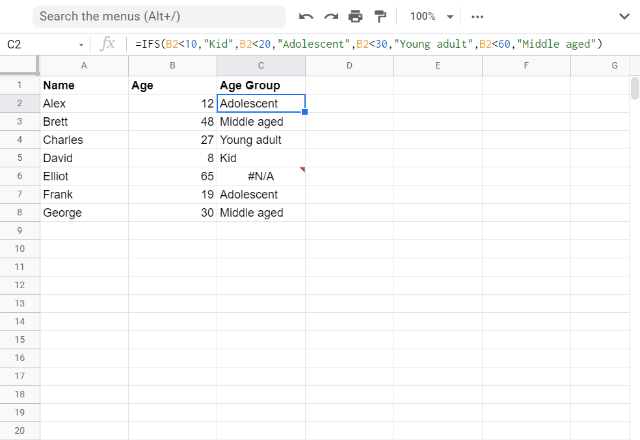The spreadsheet goes through each expression one by one and evaluates it to be TRUE or FALSE. If an expression is TRUE, it will output the value associated with that expression; if it is FALSE, the function moves on to the next expression.

As you can observe, the age group for ages beyond 60 is not defined.

For Elliot, who is 65, Google Sheets has evaluated all the expressions as FALSE and has no value to return. Hence you see the #N/A! error.

To avoid the error, you can add an expression that will evaluate as TRUE for Elliot.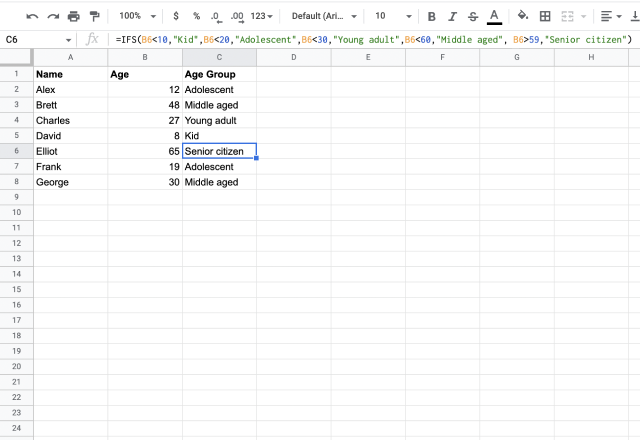Here, I’ve added a “Senior citizen” category that applies to Elliot. As you can see, the #N/A! error has been resolved.

If you were to execute the above-mentioned logic using only IF functions, that would entail creating 5th level nested IF statements, which can get very long and complex.

#### Comparison with nested IFS

In the image below, I’ve added another column where I’ve done just that. Clearly, it still works when you use an IF statement, but you can see that the function is longer and involves many more brackets, which can be confusing and lead to errors if you don’t have the correct brackets in the correct place.

So, needless to say, the IFS function is a great alternative to otherwise complex nested IF statements.I’ll now show another application of the IFS function.

The logic is the same, and I hope that after seeing these examples, you’ll have a solid understanding of how to use the IFS function, and of the different applications that it can be used for.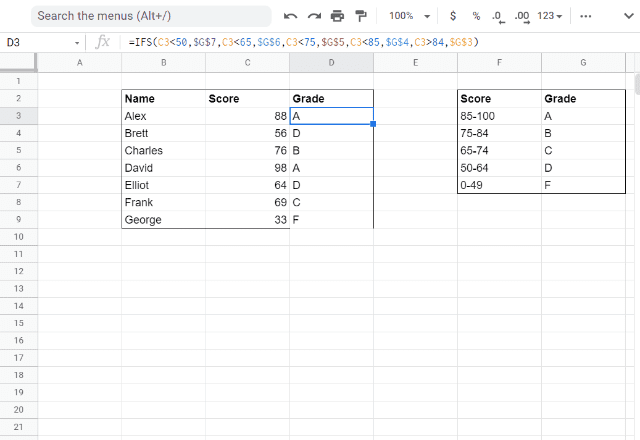Here I am assigning students a letter grade based on a test score. In the table on the right, you can see the categories of letter grades that correspond to the test scores. Take a look at the table on the left. There you can see I’ve used the IFS function to assign the grades based on these ranges.

In this case, I used the formula  =IFS(C3<50,\$G\$7,C3<65,\$G\$6,C3<75,\$G\$5,C3<85,\$G\$4,C3>84,\$G\$3).